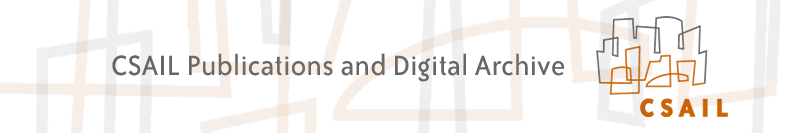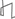Research Abstracts Home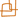CSAIL Digital Archive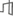Research Activities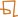CSAIL HomeResearch Abstracts - 2007IntroductionPerception & LearningPhysical, Biological & Social SystemsSystemsTheory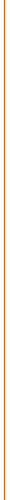### On the Hardness and Smoothed Complexity of Quasi-concave Minimization

#### Jon Kelner & Evdokia Nikolova

Concave minimization is a fundamental problem in optimization. Quasi-concave minimization is a generalization of the problem which retains the property that the minimum is attained at an extreme point of the feasible set. Despite this nice characterization of the minima, extreme points of the feasible set are typically exponentially many and even minimizing a concave quadratic function over the unit hypercube is NP-hard. In addition no efficient approximation algorithms exist to date, even for the class of low-rank quasi-concave functions, whose non-convexity lies in a low-dimensional subspace. In this paper, we provide the first smoothed analysis of quasi-concave minimization. The analysis is based on a smoothed bound for the number of extreme points of the projection of the feasible polytope onto a k-dimensional subspace, where k is the rank (informally, the dimension of nonconvexity) of the quasi-concave function. Our smoothed bound is polynomial in the original dimension of the problem n, the perturbation size and it is exponential in the rank of the function k. For low-rank functions with constant k this implies an expected polynomial time algorithm for quasi-concave minimization. We also prove (log n)-hardness of approximation of general quasi-concave minimization, which shows that our smoothed bound is tight in a sense that no polynomial smoothed bound is possible for quasi-concave functions of general rank k.Computer Science and Artificial Intelligence Laboratory (CSAIL) The Stata Center, Building 32 - 32 Vassar Street - Cambridge, MA 02139 - USA tel:+1-617-253-0073 - publications@csail.mit.edu